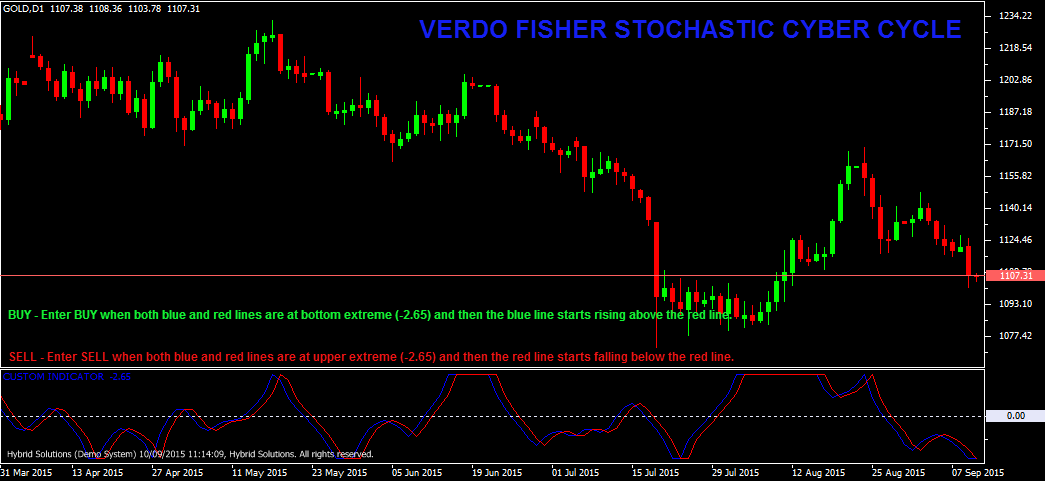# Fischer Stochastic Cyber Cycle

Free

Developed By: NeeduVerdeSolution

In stock

Fisher Stochastic Cyber Cycle is a VertexFX indicator calculated from the Cyber Cycle in three steps. In the first step we calculate the Cyber Cycle value. Then in second step stochastic are applied to this value, and finally a Fisher transform is applied to theresult. This indicator is useful to detect market cycles and turning points.

SKU: 666 Categories: ,Fisher Stochastic Cyber Cycle is a VertexFX indicator calculated from the Cyber Cycle in three steps. In the first step we calculate the Cyber Cycle value. Then in second step stochastic are applied to this value, and finally a Fisher transform is applied to theresult. This indicator is useful to detect market cycles and turning points.

Fisher Stochastic Cyber Cycle includes two indicators, Fisher Stochastic Cyber Cycle (Blue) sign and the Trigger (Red) signal. At the point when the Fisher Stochastic Cyber Cycle line crosses over the Trigger line, the market is at the bottom of the cycle. Likewise, when the Fisher Stochastic Cyber Cycle line crosses underneath the Trigger line, we are at the highest point of the cycle.

This indicator has a minimal lag of one to two bars. The median price is first smoothed over 3 bars using a Weighted Moving Average. Then this smoothed price further smoothed using Alpha to calculate the Cyber Cycle value. This value is normalized using stochastic value and weighted using (WMA) over the Length period of bars to generate the Stochastic Cyber Cycle. Finally, a Fisher transform is applied to derive the Fisher Stochastic Cyber Cycle. The Trigger line is derived from the previous bar value of the Fisher Stochastic Cyber Cycle indicator. This indicator is bounded between +2.65 and -2.65 range.

BUY – Place Buy when both blue and red lines are at bottom extreme (-2.65) and then blue line starts rising above red line.
SELL – Place Sell when both blue and red lines are at upper extreme (-2.65) and then red line starts falling below red line.